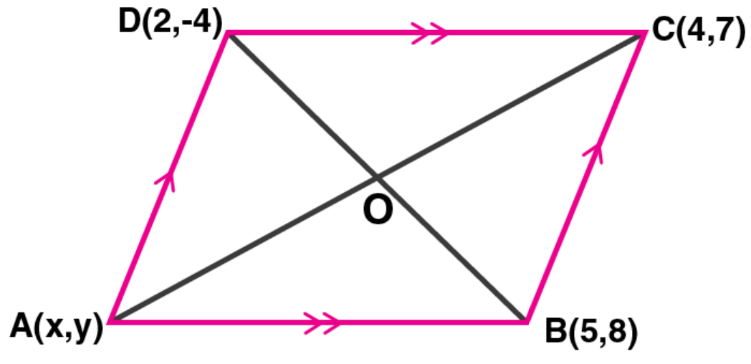Newbie

# ABCD is a parallelogram where A (x, y), B (5, 8), C (4, 7) and D (2, – 4). Find (i) the coordinates of A (ii) the equation of the diagonal BD.

• 0

An Important Question of M.L Aggarwal book of class 10 Based on Equation of a Straight Line Chapter for ICSE BOARD.
Here given that ABCD is a parallelogram where 4 coordinated are given.
So, you have to find the coordinates of A and the equation of the diagonal BD.
This is the Question Number 22, Exercise 12.1 of M.L Aggarwal.

Share

1.(i) Given,

ABCD is a parallelogram where A (x, y), B (5, 8), C (4, 7) and D (2, -4)

O in the point of intersection of the diagonals of the parallelogram.

So, the co-ordinates of O = ([5 + 2]/2, [8 – 4]/2) = (3.5, 2)

Now, for the line AC we have

3.5 = (x + 4)/2 and 2 = (y + 7)/27 = x + 4 and 4 = y + 7

x = 7 – 4 and y = 4 – 7

x = 3 and y = -3

Thus, the co-ordinates of A are (3, -3).

(ii) Equation of diagonal BD is given by

y – 8 = (-4 – 8)/(2 – 5) × (x – 5)

y – 8 = (-12/-3) × (x – 5)

y – 8 = 4 (x – 5)

y – 8 = 4x – 20

4x – y – 20 + 8 = 0

Hence, the equation of the diagonal is 4x – y – 12 = 0.

• 0